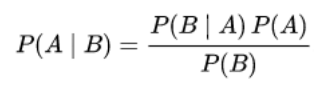# Bayes Theorem

## What is Bayes' Theorem?

Bayes’ theorem is a formula that governs how to assign a subjective degree of belief to a hypothesis and rationally update that probability with new evidence. This is the fundamental tool and methodology behind Bayesian probability, statistics and programming, as well as the second major form of probability interpretation.

Both frequentist and Bayesian probability have a role to play in machine learning. For example, if dealing with truly random and discrete variables, such as landing a six in a die roll, the traditional approach of simply calculating the odds (frequency) is the fastest way to model a likely outcome. However, if the six keeps coming up far more often than the predicated 1/6 odds, only Bayesian probability would take that new observation into account and increase the confidence level that someone is playing with loaded dice.

## How to Use Bayes’ Theorem

Bayes’ theorem can be applied in both hypothesis testing or conditional probability with the following formula:Where:

• P()  = the likelihood of event $B$occurring given that $A$is true.
•= Hypothesis or event 1
•= Evidence or event 2
• P() or P() = Prior probability of the hypothesis before seeing any evidence, as well as the marginal probability, i.e. the chance of observing A and B independent of each other.

• P() = Posterior probability of the hypothesis after updating for new evidence, as well as the conditional probability that the second event occurs if event one is true.